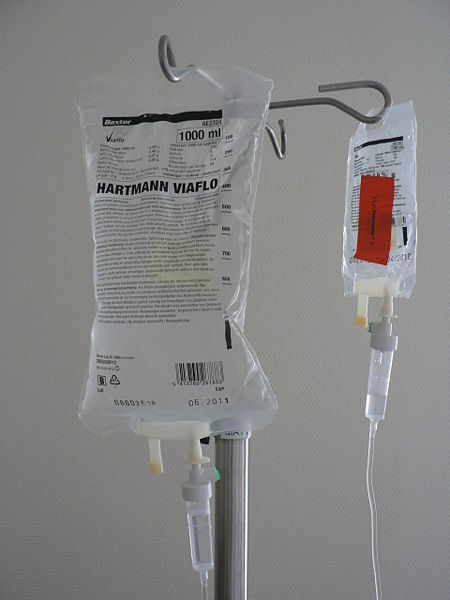## Dilutions and Concentrations

### Learning Objective

1. Learn how to dilute and concentrate solutions.

Often, a worker will need to change the concentration of a solution by changing the amount of solvent. Dilution is the addition of solvent, which decreases the concentration of the solute in the solution. Concentration is the removal of solvent, which increases the concentration of the solute in the solution. (Do not confuse the two uses of the word concentration here!)

In both dilution and concentration, the amount of solute stays the same. This gives us a way to calculate what the new solution volume must be for the desired concentration of solute. From the definition of molarity,

molarity = moles of solute / liters of solution

we can solve for the number of moles of solute:

moles of solute = (molarity)(liters of solution)

A simpler way of writing this is to use M to represent molarity and V to represent volume. So the equation becomes

moles of solute = MV

Because this quantity does not change before and after the change in concentration, the product MV must be the same before and after the concentration change. Using numbers to represent the initial and final conditions, we have

M1V1 = M2V2

as the dilution equation. The volumes must be expressed in the same units. Note that this equation gives only the initial and final conditions, not the amount of the change. The amount of change is determined by subtraction.

### Example 9

If 25.0 mL of a 2.19 M solution are diluted to 72.8 mL, what is the final concentration?

Solution

It does not matter which set of conditions is labelled 1 or 2, as long as the conditions are paired together properly. Using the dilution equation, we have

(2.19 M)(25.0 mL) = M2(72.8 mL)

Solving for the second concentration (noting that the milliliter units cancel),

M2 = 0.752 M

The concentration of the solution has decreased. In going from 25.0 mL to 72.8 mL, 72.8 − 25.0 = 47.8 mL of solvent must be added.

Test Yourself

A 0.885 M solution of KBr whose initial volume is 76.5 mL has more water added until its concentration is 0.500 M. What is the new volume of the solution?

135.4 mL

Concentrating solutions involves removing solvent. Usually this is done by evapourating or boiling, assuming that the heat of boiling does not affect the solute. The dilution equation is used in these circumstances as well.

### Chemistry Is Everywhere: Preparing IV Solutions

In a hospital emergency room, a physician orders an intravenous (IV) delivery of 100 mL of 0.5% KCl for a patient suffering from hypokalemia (low potassium levels). Does an aide run to a supply cabinet and take out an IV bag containing this concentration of KCl?

Not likely. It is more probable that the aide must make the proper solution from an IV bag of sterile solution and a more concentrated, sterile solution, called a stock solution, of KCl. The aide is expected to use a syringe to draw up some stock solution and inject it into the waiting IV bag and dilute it to the proper concentration. Thus the aide must perform a dilution calculation.Medical personnel commonly must perform dilutions for IV solutions. Source: “Infuuszakjes” by Harmid is in the public domain.

If the stock solution is 10.0% KCl and the final volume and concentration need to be 100 mL and 0.50%, respectively, then it is an easy calculation to determine how much stock solution to use:

(10%)V1 = (0.50%)(100 mL)
V1 = 5 mL

Of course, the addition of the stock solution affects the total volume of the diluted solution, but the final concentration is likely close enough even for medical purposes.

Medical and pharmaceutical personnel are constantly dealing with dosages that require concentration measurements and dilutions. It is an important responsibility: calculating the wrong dose can be useless, harmful, or even fatal!

### Key Takeaways

• Calculate the new concentration or volume for a dilution or concentration of a solution.

### Exercises

1. What is the difference between dilution and concentration?

2. What quantity remains constant when you dilute a solution?

3. A 1.88 M solution of NaCl has an initial volume of 34.5 mL. What is the final concentration of the solution if it is diluted to 134 mL?

4. A 0.664 M solution of NaCl has an initial volume of 2.55 L. What is the final concentration of the solution if it is diluted to 3.88 L?

5. If 1.00 mL of a 2.25 M H2SO4 solution needs to be diluted to 1.00 M, what will be its final volume?

6. If 12.00 L of a 6.00 M HNO3 solution needs to be diluted to 0.750 M, what will be its final volume?

7. If 665 mL of a 0.875 M KBr solution are boiled gently to concentrate the solute to 1.45 M, what will be its final volume?

8. If 1.00 L of an LiOH solution is boiled down to 164 mL and its initial concentration is 0.00555 M, what is its final concentration?

9. How much water must be added to 75.0 mL of 0.332 M FeCl3(aq) to reduce its concentration to 0.250 M?

10. How much water must be added to 1.55 L of 1.65 M Sc(NO3)3(aq) to reduce its concentration to 1.00 M?

1.

Dilution is a decrease in a solution’s concentration, whereas concentration is an increase in a solution’s concentration.

3.

0.484 M

5.

2.25 mL

7.

401 mL

9.

24.6 mL